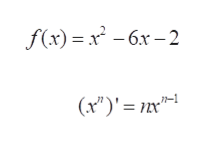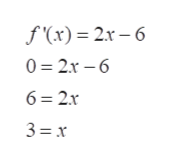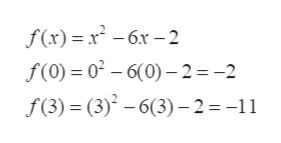Find the absolute extrema of the function on the closed interval. Use a graphing utility to verify your results. (If an answer does not exist, enter DNE.)f(x) = x2 − 6x − 2,    [0, 3]absolute maximum    (x, y) =        absolute minimum    (x, y) =

Question

Find the absolute extrema of the function on the closed interval. Use a graphing utility to verify your results. (If an answer does not exist, enter DNE.)

f(x) = x2 − 6x − 2,    [0, 3]
absolute maximum     (x, y)  =

absolute minimum     (x, y)  =

Step 1

To find absolute extrema, take derivative and check the intervals using f(x). Apply power rule to take derivativehelp_outlineImage Transcriptionclose- x* - 6х -2 -бх — 2 f(x) (x)'n т fullscreen
Step 2

Apply power rule to find derivative. Set derivative =0 and solve for xhelp_outlineImage Transcriptionclosef'(x) 2x-6 0 2x-6 6 2x 3 x fullscreen
Step 3

Critical value is x=3, Given interval is [...help_outlineImage Transcriptionclosef(x)x6x2 f(0)= 02 -6(0)-2 =-2 f(3) (3) -6(3)-2 = -11 fullscreen

Want to see the full answer?

See Solution

Want to see this answer and more?

Our solutions are written by experts, many with advanced degrees, and available 24/7

See Solution
Tagged in

Derivative